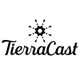# TierraCastTierraCast

#### Brass 10mm Cable Chain - Sold By 6 Inches

TierraCast

\$3.90
Sold by 6 inches times the quantity in one continuous strand - for example: if you would like one strand of chain that is 7 feet long, you would select a quantity of 14. (6 inches equals roughly...
\$3.90

#### Antique Silver 2x6mm Paperclip Cable Chain - Sold By 6 Inches

TierraCast

\$2.10
Sold by 6 inches times the quantity in one continuous strand - for example: if you would like one strand of chain that is 7 feet long, you would select a quantity of 14. (6 inches equals roughly...
\$2.10

#### Silver 6x4mm Cable Chain - Sold By 6 Inches

TierraCast

\$2.10
Sold by 6 inches times the quantity in one continuous strand - for example: if you would like one strand of chain that is 7 feet long, you would select a quantity of 14. (6 inches equals roughly...
\$2.10

#### Antique Copper 6x4mm Cable Chain - Sold By 6 Inches

TierraCast

\$2.70
Sold by 6 inches times the quantity in one continuous strand - for example: if you would like one strand of chain that is 7 feet long, you would select a quantity of 14. (6 inches equals roughly...
\$2.70

#### Gold 6x4mm Cable Chain - Sold By 6 Inches

TierraCast

\$2.40
Sold by 6 inches times the quantity in one continuous strand - for example: if you would like one strand of chain that is 7 feet long, you would select a quantity of 14. (6 inches equals roughly...
\$2.40

#### Antique Brass 6x4mm Cable Chain - Sold By 6 Inches

TierraCast

\$2.40
Sold by 6 inches times the quantity in one continuous strand - for example: if you would like one strand of chain that is 7 feet long, you would select a quantity of 14. (6 inches equals roughly...
\$2.40

#### Antique Silver 9x6mm Textured Cable Chain - Sold By 6 Inches

TierraCast

\$2.40
Sold by 6 inches times the quantity in one continuous strand - for example: if you would like one strand of chain that is 7 feet long, you would select a quantity of 14. (6 inches equals roughly...
\$2.40

#### Gold 9x6mm Textured Cable Chain - Sold By 6 Inches

TierraCast

\$3.00
Sold by 6 inches times the quantity in one continuous strand - for example: if you would like one strand of chain that is 7 feet long, you would select a quantity of 14. (6 inches equals roughly...
\$3.00

#### Antique Brass 4x2.5mm Cable Chain - Sold By 6 Inches

TierraCast

\$2.40
Sold by 6 inches times the quantity in one continuous strand - for example: if you would like one strand of chain that is 7 feet long, you would select a quantity of 14. (6 inches equals roughly...
\$2.40

#### Antique Silver 4x2.5mm Cable Chain - Sold By 6 Inches

TierraCast

\$2.10
Sold by 6 inches times the quantity in one continuous strand - for example: if you would like one strand of chain that is 7 feet long, you would select a quantity of 14. (6 inches equals roughly...
\$2.10

#### Gold Plated 6x3.5mm Ladder Chain - Sold By 6 Inches

TierraCast

\$2.40
Sold by 6 inches times the quantity in one continuous strand - for example: if you would like one strand of chain that is 7 feet long, you would select a quantity of 14. (6 inches equals roughly...
\$2.40

#### Antique Silver Plated 6x3.5mm Ladder Chain - Sold By 6 Inches

TierraCast

\$2.10
Sold by 6 inches times the quantity in one continuous strand - for example: if you would like one strand of chain that is 7 feet long, you would select a quantity of 14. (6 inches equals roughly...
\$2.10

#### Imitation Rhodium Plated 6x3.5mm Ladder Chain - Sold By 6 Inches

TierraCast

\$3.00
Sold by 6 inches times the quantity in one continuous strand - for example: if you would like one strand of chain that is 7 feet long, you would select a quantity of 14. (6 inches equals roughly...
\$3.00

#### Immitation Rhodium Plated 5x6mm Cable Chain - Sold By 6 Inches

TierraCast

\$3.00
Sold by 6 inches times the quantity in one continuous strand - for example: if you would like one strand of chain that is 7 feet long, you would select a quantity of 14. (6 inches equals roughly...
\$3.00